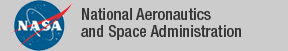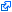### NASA PVS Library

The NASA PVS Library is a collection of formal developments in PVS maintained by the NASA Langley Formal Methods Team and is part of the PVS research sponsored by NASA Langley.

The current version of the library is available from GitHub.

### Contributions

• Affine Arithmetic: Formalization of affine arithmetic and strategy for evaluating polynomial functions with variables on interval domains (examples).
• Bernstein: Formalization of multivariate Bernstein polynomials.
• Hypatheon: A database capability for PVS theories.
• Interval Arithmetic: Formalization of interval arithmetic and strategies based on intrerval analysis (examples and more examples).
• MetiTarski/Z3: Integration of the theorem prover MetiTarskiand SMT solver Z3as external oracles to PVS (examples).
• Sturm: Formalization of Sturm's Theorem and strategies for proving univariate polynomial relations over a real interval (examples).
• Tarski: Formalization of Tarski's Theorem and strategy for proving systems of univariate polynomial relations on the real line (examples).
• Pre-installed in PVS:
• PVSio: Animation tool of PVS functional specifications (examples).

### Previous versions of the NASA PVS Library

The tagidentifies links that are outside the NASA domain